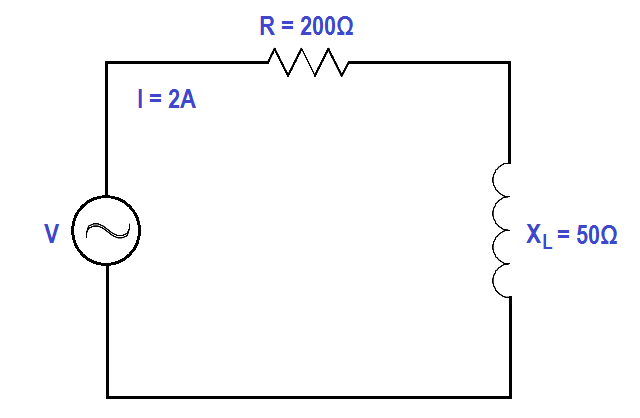# Calculate Power in Series RL Circuit

A 200 Ω resistor and a 50 Ω XL are placed in series with a voltage source, and the total current flow is 2 amps, as shown in Figure.Figure : Series R-L Circuit

Find:

1. Power Factor, pf
2. Applied voltage, V
3. True Power, P
4. Reactive Power, Q
5. Apparent Power, S

Solution:

##### 1. Calculate Power factor (pf)p.f. = cos (14º)

p.f. = 0.097

##### 2. Calculate Applied Voltage, V

V = I Z

Z = √R2 + XL2

so, V = I√R2 + XL2

V = 2√2002 + 502

V = 2√42500

V = 2 x 206.16

V = 412.3 Volts

##### 3. Calculate True Power, P

P = EI cos θ

P = (412.3)(2)(0.97)

P = 799.86 watts

##### 4. Calculate Reactive Power, Q

Q = EI sin θ

Q = (412.3)(2)(0.242)

Q = 199.6 VAR

S = EI

S = (412.3)(2)

S = 824.6 VA